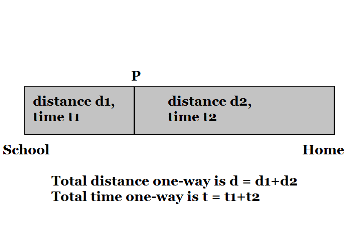# SolutionWell, this is how I worked it out; please let me know if I did it right.

Suppose, the distance between Swapnil's home and the school is "d" and he takes "t" minutes to ride this distance. So, every day he rides a distance "d" to school in time "t" and, then, rides a distance "d" back home in time "t". So, he travels a distance 2*d in time 2*t every day. On this particular Saturday, he did not ride right up to the school but met his son somewhere on the way. Let's suppose he met his son at point "P". Let's suppose the distance between Swapnil's house and "P" is "d2" and it takes him time "t2" to cover that distance, and that the distance between "P" and the school is "d1" and it takes Swapnil time "t1" to cover that distance. Thus, d = d1 + d2 and t = t1 + t2.

But, on this Saturday, Swapnil rode only up to "P" and returned; so, the distance he travelled was 2*d2; the time he took was 2*t2. This time was 30 minutes less than on any other day. Therefore 2*t2 = 2*t - 30.

Now, t = t1 + t2 as already mentioned. So, 2*t2 = 2*(t1 + t2) - 30.

So, 2*t2 = 2*t1 + 2*t2 - 30.

So, 2*t1 = 30.

So, t1 = 15 minutes.

But, what is t1? It is the time required by Swapnil to travel the distance between "P" and the school. So, if he decides to reach the school at exactly 5pm, he must reach "P" at 4.45pm. Therefore, Swapnil reaches "P" at 4.45pm where he meets his son. The son had started walking at 4pm and reached "P" at 4.45 pm. So, the poor boy had walked for 45 minutes.

• Union Public Service Commission - www.upsc.gov.in
• IIT-Kharagpur - www.iitkgp.ac.in
• Indian Statistical Institute - www.isical.ac.in
• Indian Institute of Technology Madras - www.iitm.ac.in
• Indian Institute of Management, Ahmedabad - www.iimahd.ernet.in
• Indian Institute of Mass Commission - www.iimc.nic.in
• IIT Bombay - www.iitb.ac.in